Courses
Courses for Kids
Free study material
Offline Centres
More

# NCERT Solutions for Class 10 Maths Chapter 11 - ExerciseLast updated date: 02nd Dec 2023
Total views: 531.9k
Views today: 12.31k

## NCERT Solutions for Class 10 Maths Chapter 11 Constructions (Ex 11.1) Exercise 11.1

Important Topics under NCERT Solutions for Class 10 Maths Chapter 11 Constructions Ex 11.1

NCERT Class 10 Maths Chapter 11 syllabus is on Constructions. It is a very important chapter covered in class 10 and is divided into 4 main topics. The following is a table containing the important topics that come under NCERT Class 10 Maths Chapter 11 Constructions, and we recommend that students pay close attention to these topics to reap the benefits of the solutions provided by Vedantu for this chapter.

 Sl. No. Topic 1. Introduction 2. Division of a Line Segment 3. Construction of Tangents to a Circle 4. Summary

We advise students to make full use of the solutions that we have provided for this chapter on constructions to be able to score desirable marks in their exams, and going through these 4 important subtopics will help them in practising the given solutions with ease.

Backup

Free PDF download of NCERT Solutions for Class 10 Maths Chapter 11 Exercise 11.1 (Ex 11.1) and all chapter exercises at one place prepared by expert teacher as per NCERT (CBSE) books guidelines. Class 10 Maths NCERT Solutions Chapter 11 Constructions Exercise 11.1 Questions with Solutions to help you to revise complete Syllabus and Score More marks. Register and get all exercise CBSE Solutions in your emails. Subjects like Science, Maths, English will become easy to study if you have access to Class 10 Science NCERT Solutions, Maths solutions, and solutions of other subjects that are available on Vedantu only.

Important Topics under NCERT Solutions for Class 10 Maths Chapter 11 Constructions Ex 11.1

NCERT Class 10 Maths Chapter 11 syllabus is on Constructions. It is a very important chapter covered in class 10 and is divided into 4 main topics. The following is a table containing the important topics that come under NCERT Class 10 Maths Chapter 11 Constructions, and we recommend that students pay close attention to these topics to reap the benefits of the solutions provided by Vedantu for this chapter.

 Sl. No. Topic 1. Introduction 2. Division of a Line Segment 3. Construction of Tangents to a Circle 4. Summary

We advise students to make full use of the solutions that we have provided for this chapter on constructions to be able to score desirable marks in their exams, and going through these 4 important subtopics will help them in practising the given solutions with ease.

## Access NCERT Solutions for Class 10 Maths Chapter 11 – Construction

Exercise 11.1

1. Draw a line segment of length $7.6$ cm and divide it in the ratio $5:8$. Measure the two parts. Give the justification of the construction.

Ans: To divide a line segment of length $7.6$ cm in the ratio of $5:8$ follow the steps below:

Step 1: Draw line segment $AB$ of $7.6$

Step 2: Draw a ray $AX$ making an acute angle with line segment $AB$.

Step 3: Mark $13$ points ${{A}_{1}},{{A}_{2}},...,{{A}_{13}}$ on $AX$such that the length of each segment is equal.

Step 4: Join $B$ with ${{A}_{13}}$.

Step 5: Draw a line parallel to $B{{A}_{13}}$ passing through ${{A}_{5}}$ and intersecting $AB$ at point $C$.

The point $C$ divides the line segment $AB$ in the ratio of $5:8$. The length of required segments are: $AC=2.9cm$ and $BC=4.7cm$.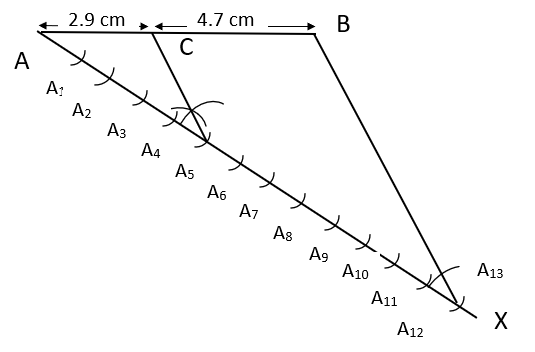Justification

By construction, we have $\vartriangle A{{A}_{5}}C,\vartriangle AB{{A}_{13}}$ with ${{A}_{5}}C\parallel B{{A}_{13}}$ and vertex $A$  common. Therefore,

$\dfrac{AC}{BC}=\dfrac{A{{A}_{5}}}{{{A}_{5}}{{A}_{13}}}$

Since the distance between ${{A}_{i}},{{A}_{i+1}}$ are equal. Therefore,

$\dfrac{A{{A}_{5}}}{{{A}_{5}}{{A}_{13}}}=\dfrac{5}{13-5}=\dfrac{5}{8}$

Hence, the point $C$ divides the line segment $AB$ in the ratio of $5:8$.

2. Construct a triangle of sides $4$ cm, $5$ cm and $6$ cm and then a triangle similar to it whose sides are $\dfrac{2}{3}$ of the corresponding sides of the first triangle. Give the justification of the construction.

Ans: To construct the required triangle, follow the steps below:

Step 1: Draw a line segment $\text{AB}$ of length $4$ cm.

Step 2: Now, take point $A$ as a centre and draw an arc of $5$ cm radius.

Step 3: Now, take point $B$ as a centre and draw an arc of $6$ cm radius.

Step 4: The point at which the arcs from step 3 and 2 meet. Name it as $C$. $\vartriangle ABC$ is the required triangle.

Step 5: Draw a ray $AX$ on the opposite side of vertex $C$ making an acute angle with line $AB$.

Step 6: Mark $3$ points ${{A}_{1}},{{A}_{2}},{{A}_{3}}$ on $AX$ such that the length of each segment is equal.

Step 7: Join $B$ with ${{A}_{3}}$.

Step 8: Draw a line parallel to $B{{A}_{3}}$ passing through ${{A}_{2}}$ and intersecting $AB$ at point $B'$.

Step 9: Draw a line parallel to $BC$ passing through $B'$ and intersecting $AC$ at point $C'$.

$\vartriangle AB'C'$ is the required triangle.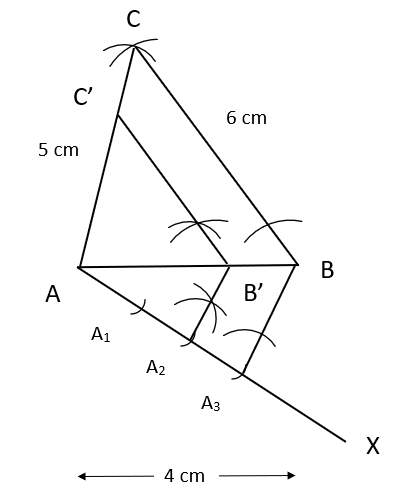Justification

By construction, we have $\vartriangle ABC,\vartriangle AB'C'$ with $BC\parallel B'C'$ and vertex $A$  common. Therefore, by corresponding angle property, $\angle ABC=\angle AB'C'$

Hence, by  $AA$ similarity criteria, $\vartriangle ABC\sim \vartriangle AB'C'$

$\Rightarrow \dfrac{AB'}{AB}=\dfrac{B'C'}{BC}=\dfrac{AC'}{AC}$  ….. (1)

Consider triangles, $\vartriangle A{{A}_{2}}B',\vartriangle A{{A}_{3}}B$ with ${{A}_{2}}B'\parallel {{A}_{3}}B$ and vertex $A$  common. Therefore, by corresponding angle property, $\angle A{{A}_{2}}B'=\angle A{{A}_{3}}B$

Hence, by  $AA$ similarity criteria, $\vartriangle A{{A}_{2}}B'\sim \vartriangle A{{A}_{3}}B$

$\Rightarrow \dfrac{AB'}{AB}=\dfrac{A{{A}_{2}}}{A{{A}_{3}}}=\dfrac{2}{3}$  ….. (2)

Hence from (1) and (2),

$\Rightarrow AB'=\dfrac{2}{3}AB,\text{ }AC'=\dfrac{2}{3}AC\text{ and }B'C'=\dfrac{2}{3}BC$

3. Construct a triangle with sides $5$ cm, $6$ cm and $7$ cm and then another triangle whose sides are $\dfrac{7}{5}$ of the corresponding sides of the first triangle. Give the justification of the construction.

Ans: To construct the required triangle, follow the steps below:

Step 1: Draw a line segment $\text{AB}$ of length $5$ cm and extend it from $B$ end.

Step 2: Now, take point $A$ as a centre and draw an arc of $6$ cm radius.

Step 3: Now, take point $B$ as a centre and draw an arc of $7$ cm radius.

Step 4: The point at which the arcs from step 3 and 2 meet. Name it as $C$. $\vartriangle ABC$ is the required triangle.

Step 5: Draw a ray $AX$ on the opposite side of vertex $C$ making an acute angle with line $AB$.

Step 6: Mark $7$ points ${{A}_{1}},{{A}_{2}},...,{{A}_{7}}$ on $AX$such that the length of each segment is equal.

Step 7: Join $B$ with ${{A}_{5}}$.

Step 8: Draw a line parallel to $B{{A}_{5}}$ passing through ${{A}_{7}}$ and intersecting extended $AB$ at point $B'$.

Step 9: Draw a line parallel to $BC$ passing through $B'$ and intersecting extended $AC$ at point $C'$.

$\vartriangle AB'C'$ is the required triangle.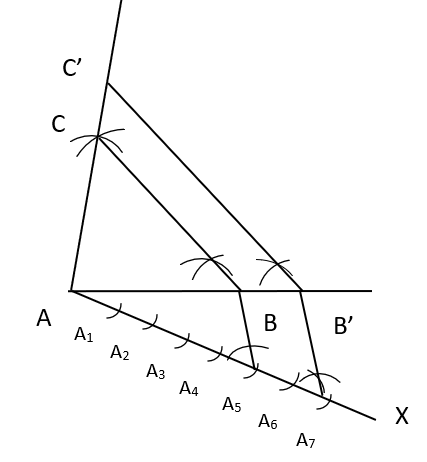Justification

By construction, we have $\vartriangle ABC,\vartriangle AB'C'$ with $BC\parallel B'C'$ and vertex $A$  common. Therefore, by corresponding angle property, $\angle ABC=\angle AB'C'$

Hence, by  $AA$ similarity criteria, $\vartriangle ABC\sim \vartriangle AB'C'$

$\Rightarrow \dfrac{AB'}{AB}=\dfrac{B'C'}{BC}=\dfrac{AC'}{AC}$  ….. (1)

Consider triangles, $\vartriangle A{{A}_{7}}B',\vartriangle A{{A}_{5}}B$ with ${{A}_{7}}B'\parallel {{A}_{5}}B$ and vertex $A$  common. Therefore, by corresponding angle property, $\angle A{{A}_{7}}B'=\angle A{{A}_{5}}B$

Hence, by  $AA$ similarity criteria, $\vartriangle A{{A}_{7}}B'\sim \vartriangle A{{A}_{5}}B$

$\Rightarrow \dfrac{AB'}{AB}=\dfrac{A{{A}_{7}}}{A{{A}_{5}}}=\dfrac{7}{5}$  ….. (2)

Hence from (1) and (2),

$\Rightarrow AB'=\dfrac{7}{5}AB,\text{ }AC'=\dfrac{7}{5}AC\text{ and }B'C'=\dfrac{7}{5}BC$

4. Construct an isosceles triangle whose base is $8$ cm and altitude $4$ cm and then another triangle whose side are $1\dfrac{1}{2}$ times the corresponding sides of the isosceles triangle. Give the justification of the construction.

Ans: To construct the required triangle, follow the steps below:

Step 1: Draw a line segment $\text{AB}$ of length $8$ cm and extend it from $B$ end.

Step 2: Now, take point $A$ and $B$ as centres and draw arcs of $6$ cm radius. The point at which the arcs meet is $O,O'$. Join the line $OO'$.

Step 3: Let the line $OO'$ meet the line $AB$ at $D$.  Now, take point $D$ as centre and draw an arc of $4$ cm radius.

Step 4: Let the arc cuts the line $OO'$ at point $C$. $\vartriangle ABC$ is the required isosceles triangle.

Step 5: Draw a ray $AX$ on the opposite side of vertex $C$ making an acute angle with line $AB$.

Step 6: Mark $3$ points ${{A}_{1}},{{A}_{2}},{{A}_{3}}$ on $AX$such that the length of each segment is equal.

Step 7: Join $B$ with ${{A}_{2}}$.

Step 8: Draw a line parallel to $B{{A}_{2}}$ passing through ${{A}_{3}}$ and intersecting extended $AB$ at point $B'$.

Step 9: Draw a line parallel to $BC$ passing through $B'$ and intersecting extended $AC$ at point $C'$.

$\vartriangle AB'C'$ is the required triangle.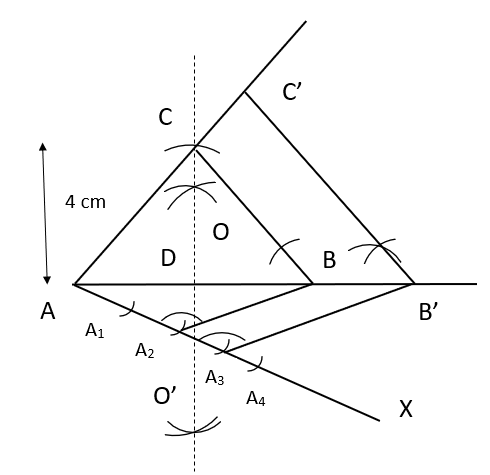Justification

By construction, we have $\vartriangle ABC,\vartriangle AB'C'$ with $BC\parallel B'C'$ and vertex $A$  common. Therefore, by corresponding angle property, $\angle ABC=\angle AB'C'$

Hence, by  $AA$ similarity criteria, $\vartriangle ABC\sim \vartriangle AB'C'$

$\Rightarrow \dfrac{AB'}{AB}=\dfrac{B'C'}{BC}=\dfrac{AC'}{AC}$  ….. (1)

Consider triangles, $\vartriangle A{{A}_{3}}B',\vartriangle A{{A}_{2}}B$ with ${{A}_{3}}B'\parallel {{A}_{2}}B$ and vertex $A$  common. Therefore, by corresponding angle property, $\angle A{{A}_{3}}B'=\angle A{{A}_{2}}B$

Hence, by  $AA$ similarity criteria, $\vartriangle A{{A}_{3}}B'\sim \vartriangle A{{A}_{2}}B$

$\Rightarrow \dfrac{AB'}{AB}=\dfrac{A{{A}_{3}}}{A{{A}_{2}}}=\dfrac{3}{2}$  ….. (2)

Hence from (1) and (2),

$\Rightarrow AB'=\dfrac{3}{2}AB,\text{ }AC'=\dfrac{3}{2}AC\text{ and }B'C'=\dfrac{3}{2}BC$

5. Draw a triangle $\mathbf{ABC}$ with side $BC=6$ cm, $\mathbf{AB}=\mathbf{5}$ cm and $\angle ABC={{60}^{\circ }}$ . Then construct a triangle whose sides are $\dfrac{3}{4}$ of the corresponding sides of the triangle $\mathbf{ABC}$. Give the justification of the construction.

Ans: To construct the required triangle, follow the steps below:

Step 1: Draw a line segment $\text{BC}$ of length $6$ cm.

Step 2: Now, take point $B$ as centre and draw an arc of $5$ cm radius making an angle of ${{60}^{\circ }}$ with $BC$. Let the point be known as $A$

Step 3: Join $AC$. $\vartriangle ABC$ is the required triangle.

Step 4: Draw a ray $BX$ on the opposite side of vertex $A$ making an acute angle with line $BC$.

Step 5: Mark $4$ points ${{B}_{1}},{{B}_{2}},{{B}_{3}},{{B}_{4}}$ on $BX$such that the length of each segment is equal.

Step 6: Join $C$ with ${{B}_{4}}$.

Step 7: Draw a line parallel to $C{{B}_{4}}$ passing through ${{B}_{3}}$ and intersecting $BC$ at point $C'$.

Step 8: Draw a line parallel to $AC$ passing through $C'$ and intersecting $AB$ at point $B'$.

$\vartriangle AB'C'$ is the required triangle.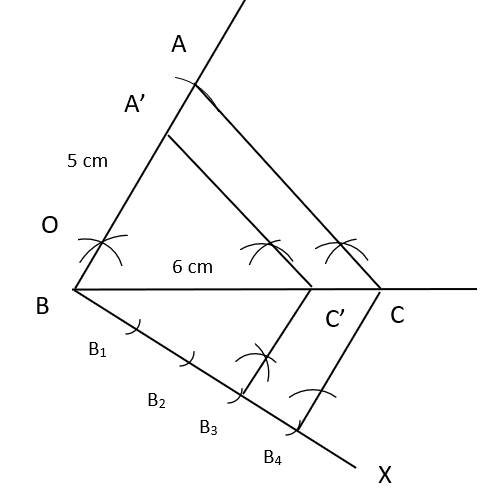Justification

By construction, we have $\vartriangle ABC,\vartriangle AB'C'$ with $AC\parallel A'C'$ and vertex $B$  common. Therefore, by corresponding angle property, $\angle BAC=\angle BA'C'$

Hence, by  $AA$ similarity criteria, $\vartriangle ABC\sim \vartriangle AB'C'$

$\Rightarrow \dfrac{AB'}{AB}=\dfrac{B'C'}{BC}=\dfrac{AC'}{AC}$  ….. (1)

Consider triangles, $\vartriangle B{{B}_{3}}C',\vartriangle B{{B}_{4}}C$ with ${{B}_{4}}C\parallel {{B}_{3}}C'$ and vertex $B$ common. Therefore, by corresponding angle property, $\angle B{{B}_{4}}C=\angle B{{B}_{3}}C'$

Hence, by  $AA$ similarity criteria, $\vartriangle B{{B}_{3}}C'\sim \vartriangle B{{B}_{4}}C$

$\Rightarrow \dfrac{BC'}{BC}=\dfrac{B{{B}_{3}}}{B{{B}_{4}}}=\dfrac{3}{4}$  ….. (2)

Hence from (1) and (2),

$\Rightarrow AB'=\dfrac{3}{4}AB,\text{ }AC'=\dfrac{3}{4}AC\text{ and }B'C'=\dfrac{3}{4}BC$

6. Draw a triangle $\mathbf{ABC}$ with side $\mathbf{BC}=\mathbf{7}$ cm,  $\angle B={{45}^{\circ }}$, $\angle A={{105}^{\circ }}$. Then, construct a triangle whose sides are $\dfrac{4}{3}$ times the corresponding side of $\vartriangle ABC$. Give the justification of the construction.

Ans: We know that sum of interior angles of a triangle is ${{180}^{\circ }}$. Therefore,

$\angle A+\angle B+\angle C={{180}^{\circ }}$

$\Rightarrow {{105}^{\circ }}+{{45}^{\circ }}+\angle C={{180}^{\circ }}$

$\therefore \angle C={{30}^{\circ }}$

To construct the required triangle, follow the steps below:

Step 1: Draw a line segment $\text{BC}$ of length $7$ cm and extend it from end $C$.

Step 2: Now, take point $B$ as centre and draw an arc making an angle of ${{45}^{\circ }}$ with $BC$.

Step 3: Now, take point $C$ as centre and draw an arc making an angle of ${{30}^{\circ }}$ with $BC$.

Step 4: Let the point where the arcs from step 2 and 3 meet be known as $A$. $\vartriangle ABC$ is the required triangle.

Step 5: Draw a ray $BX$ on the opposite side of vertex $A$ making an acute angle with line $BC$.

Step 6: Mark $4$ points ${{B}_{1}},{{B}_{2}},{{B}_{3}},{{B}_{4}}$ on $BX$such that the length of each segment is equal.

Step 7: Join $C$ with ${{B}_{3}}$.

Step 8: Draw a line parallel to $C{{B}_{3}}$ passing through ${{B}_{4}}$ and intersecting $BC$ at point $C'$.

Step 9: Draw a line parallel to $AC$ passing through $C'$ and intersecting $AB$ at point $B'$.

$\vartriangle AB'C'$ is the required triangle.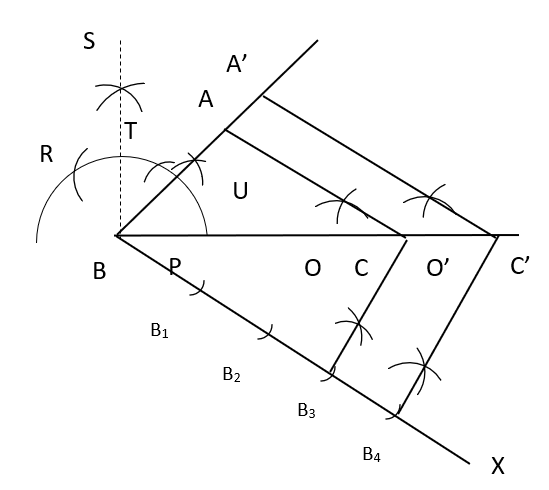Justification

By construction, we have $\vartriangle ABC,\vartriangle AB'C'$ with $AC\parallel A'C'$ and vertex $B$  common. Therefore, by corresponding angle property, $\angle BAC=\angle BA'C'$

Hence, by  $AA$ similarity criteria, $\vartriangle ABC\sim \vartriangle AB'C'$

$\Rightarrow \dfrac{AB'}{AB}=\dfrac{B'C'}{BC}=\dfrac{AC'}{AC}$  ….. (1)

Consider triangles, $\vartriangle B{{B}_{3}}C,\vartriangle B{{B}_{4}}C'$ with ${{B}_{4}}C'\parallel {{B}_{3}}C$ and vertex $B$ common. Therefore, by corresponding angle property, $\angle B{{B}_{4}}C'=\angle B{{B}_{3}}C$

Hence, by  $AA$ similarity criteria, $\vartriangle B{{B}_{3}}C\sim \vartriangle B{{B}_{4}}C'$

$\Rightarrow \dfrac{BC'}{BC}=\dfrac{B{{B}_{4}}}{B{{B}_{3}}}=\dfrac{4}{3}$  ….. (2)

Hence from (1) and (2),

$\Rightarrow AB'=\dfrac{4}{3}AB,\text{ }AC'=\dfrac{4}{3}AC\text{ and }B'C'=\dfrac{4}{3}BC$

7. Draw a right triangle in which the sides (other than hypotenuse) are of lengths $4$ cm and $3$ cm. then construct another triangle whose sides are $\dfrac{5}{3}$ times the corresponding sides of the given triangle. Give the justification of the construction.

Ans: To construct the required triangle, follow the steps below:

Step 1: Draw a line segment $\text{AB}$ of length $4$ cm and extend it from $B$ end.

Step 2: Now, take point $A$ as centres and draw an arc of $3$ cm radius and making an angle of ${{90}^{\circ }}$ with $AB$. Let the point be known as $C$.

Step 3: Join $BC$. $\vartriangle ABC$ is the required isosceles triangle.

Step 5: Draw a ray $AX$ on the opposite side of vertex $C$ making an acute angle with line $AB$.

Step 6: Mark $5$ points ${{A}_{1}},{{A}_{2}},...,{{A}_{5}}$ on $AX$such that the length of each segment is equal.

Step 7: Join $B$ with ${{A}_{3}}$.

Step 8: Draw a line parallel to $B{{A}_{3}}$ passing through ${{A}_{5}}$ and intersecting extended $AB$ at point $B'$.

Step 9: Draw a line parallel to $BC$ passing through $B'$ and intersecting extended $AC$ at point $C'$.

$\vartriangle AB'C'$ is the required triangle.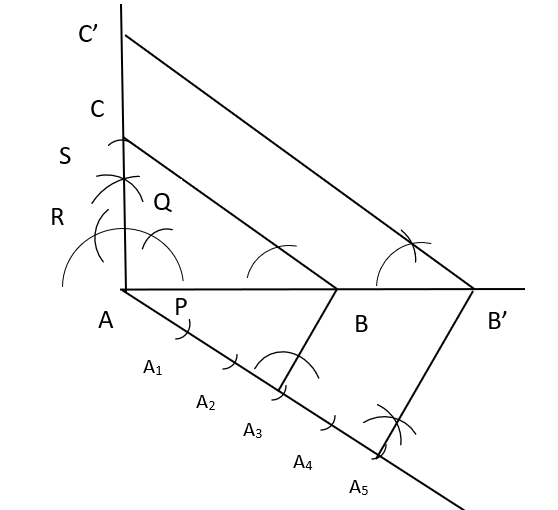Justification

By construction, we have $\vartriangle ABC,\vartriangle AB'C'$ with $BC\parallel B'C'$ and vertex $A$  common. Therefore, by corresponding angle property, $\angle ABC=\angle AB'C'$

Hence, by  $AA$ similarity criteria, $\vartriangle ABC\sim \vartriangle AB'C'$

$\Rightarrow \dfrac{AB'}{AB}=\dfrac{B'C'}{BC}=\dfrac{AC'}{AC}$  ….. (1)

Consider triangles, $\vartriangle A{{A}_{3}}B,\vartriangle A{{A}_{5}}B'$ with ${{A}_{3}}B\parallel {{A}_{5}}B'$ and vertex $A$ common. Therefore, by corresponding angle property, $\angle A{{A}_{3}}B=\angle A{{A}_{5}}B'$

Hence, by  $AA$ similarity criteria, $\vartriangle A{{A}_{3}}B\sim \vartriangle A{{A}_{5}}B'$

$\Rightarrow \dfrac{AB'}{AB}=\dfrac{A{{A}_{5}}}{A{{A}_{3}}}=\dfrac{5}{3}$  ….. (2)

Hence from (1) and (2),

$\Rightarrow AB'=\dfrac{5}{3}AB,\text{ }AC'=\dfrac{5}{3}AC\text{ and }B'C'=\dfrac{5}{3}BC$.

## NCERT Solutions for Class 10 Maths Chapter 11 Constructions (Ex 11.1) Exercise 11.1

Opting for the NCERT solutions for Ex 11.1 Class 10 Maths is considered as the best option for the CBSE students when it comes to exam preparation. This chapter consists of many exercises. Out of which we have provided the Exercise 11.1 Class 10 Maths NCERT solutions on this page in PDF format. You can download this solution as per your convenience or you can study it directly from our website/ app online.

Vedantu in-house subject matter experts have solved the problems/ questions from the exercise with the utmost care and by following all the guidelines by CBSE. Class 10 students who are thorough with all the concepts from the Maths textbook and quite well-versed with all the problems from the exercises given in it, then any student can easily score the highest possible marks in the final exam. With the help of this Class 10 Maths Chapter 11 Exercise 11.1 solutions, students can easily understand the pattern of questions that can be asked in the exam from this chapter and also learn the marks weightage of the chapter. So that they can prepare themselves accordingly for the final exam.

Besides these NCERT solutions for Class 10 Maths Chapter 11 Exercise 11.1, there are plenty of exercises in this chapter which contain innumerable questions as well. All these questions are solved/answered by our in-house subject experts as mentioned earlier. Hence all of these are bound to be of superior quality and anyone can refer to these during the time of exam preparation. In order to score the best possible marks in the class, it is really important to understand all the concepts of the textbooks and solve the problems from the exercises given next to it.

Do not delay any more. Download the NCERT solutions for Class 10 Maths Chapter 11 Exercise 11.1 from Vedantu website now for better exam preparation. If you have the Vedantu app in your phone, you can download the same through the app as well. The best part of these solutions is these can be accessed both online and offline as well.

### NCERT Solutions for Class 10 Maths PDFs (Chapter-wise)

Along with this, students can also download additional study materials provided by Vedantu, for Chapter 10 of CBSE Class 10 Maths Solutions –

## FAQs on NCERT Solutions for Class 10 Maths Chapter 11 - Exercise

1. What is Class 10 Maths Chapter 11 all about?

NCERT Class 10 Chapter 11-Constructions is a part of Geometry. This chapter is all about the construction of line segments, division of a Line Segment and Construction of a Circle, Constructions of Tangents to a circle using an analytical approach. The chapter contains four sub-topics as given in the following.

• Introduction.

• Division of a Line Segment.

• Construction of Tangents to a Circle.

• Summary.

This chapter also contains two exercises at the end which include various types and patterns of questions/ problems.

2. How many questions are there in Class 10 Maths Chapter 11 Exercise 11.1?

A total of seven questions are there in Class 10 Maths Chapter 11 Exercise 11.1 of the NCERT textbook. Answers to those problems are also provided in the NCERT Solutions of Class 10 Maths Chapter 11 provided by Vedantu. These NCERT solutions are absolutely accurate as these are created by the experienced Maths experts while adhering to the CBSE curriculum strictly.

3. Why are NCERT Solutions for Maths Chapter 11 beneficial for all the students of Class 11?

NCERT Solutions for Class 10 Maths Chapter 11 Constructions are designed in a detailed manner, where one can find a step-by-step solution to all the problems/questions for quick and easy revisions. Solutions for Chapter 11 of NCERT Class 10 Maths are prepared by the industry’s subject matter experts by following the strict guidelines of CBSE.

You can get a free PDF of NCERT Solutions for Class 10 Maths, Chapter 11 – Constructions on Vedantu site and app. Download the solutions to all the questions of NCERT exercises along with the required diagrams with a step-by-step procedure. NCERT Solutions help students in boosting their concepts and clear doubts. With the help of these NCERT Solutions for Maths Chapter 11, you will be able to understand the pattern of questions that you are going to encounter in the final exam. Not only that but also you will be able to understand how to solve these problems quickly with the help of our solutions PDF.

4. How to download NCERT Solutions for Class 10 Maths Chapter 11 Exercise 11.1?

It is very easy to download the NCERT Solutions for Class 10 Maths Chapter 11 Exercise 11.1 online. All you have to do is visit the official website of Vedantu (vedantu.com) and register yourself along with the email ID and phone number. Then you have to look for your preferred study materials such as NCERT Solutions for Class 10 Maths Chapter 11 Exercise 11.1 in this case. Once you find the page, download the PDF given on that page. Our PDFs are provided in such a way that these can be used online and offline as well.

5. What concepts does Chapter 11 of Class 10 Maths contain?

The following topics are included in Chapter 11 of Class 10 Maths:

• Introduction

• Division Of A Line Segment

• Construction Of Tangents To A Circle.

• Summary

The above-mentioned topics are explained in detail in the NCERT Maths book of Class 10. There are exercises and examples related to these points in the NCERT book. Students should solve these questions to understand the chapter. The solutions to all these questions are available on Vedantu's app and the website.

6. Is it possible to make a productive study plan for studying Chapter 11 of Class 10 Maths?

Yes, it is possible. If students want to make a better study plan for preparing Chapter 11 of Class 10 Maths, then they must follow the given steps:

• Prepare a schedule so that you can concentrate on Maths subject too.

• Understand the theory behind every topic of Chapter 11 of Class 10 Maths.

• Solve each example and question-related to the given topics.

• While solving questions of construction, remember to write the steps of construction.

• Do not use a pen while constructing the diagrams.

• Practice extra questions also so that you can have a strong grip over the chapter.

7. Which teaching aids should be used by students to practice Chapter 11 of Class 10 Maths?

The best educational materials will help students to study Chapter 11 of Class 10 Maths. The NCERT book is one of the best study materials on which students should concentrate. This book is based on the Class 10 Maths syllabus deduced by the CBSE. There are lots of questions in this book for practising. Vedantu also helps students in their studies. Students can find all the NCERT Solutions free of cost on the website of Vedantu in a more detailed and comprehensive manner. For a better understanding of the chapter, students can make use of this as a reference book.

8. Is Chapter 11 of Class 10 Maths tough or simple?

Students of Class 10 have to give Board Examinations conducted by the CBSE. They have to perform well to get good results in the Class 10 Maths Board Examination. The construction chapter may create problems while preparing for the exam. To make this chapter easy, focus on the NCERT book. Go thoroughly through each example and question to understand the chapter. Practice previous years question papers and sample papers to get an idea about exam patterns and the type of questions asked.

9. What is the summary behind every topic covered in Chapter 11 of Class 10 Maths?

• Division of a Line Segment – In this topic students will learn to divide a line segment in a given ratio by using a pencil and compass (construction). This method is used when you are not allowed to use measuring instruments.

This idea is used to construct a triangle. Both the smaller and larger triangles can be constructed.

• Construction of Tangents to a Circle –Students will learn to draw tangents with the help of the construction in this topic. The tangents will be drawn through the point present in the circle. The centre of a circle plays an important role in constructing a tangent.